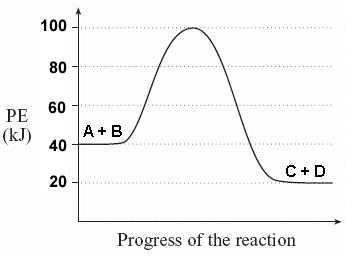# Problem: For the following reaction, answer the following questions: a. Estimate the activation energy (provide both value and unit) b. Does the graph represent an endothermic or exothermic reaction? c. Determine the enthalpy (ΔH) for the reaction product.

###### Problem Details

For the following reaction, answer the following questions:

a. Estimate the activation energy (provide both value and unit)

b. Does the graph represent an endothermic or exothermic reaction?

c. Determine the enthalpy (ΔH) for the reaction product.